Updating search results...

# 13 Results

View
Selected filters:
• UT.MATH.III.F.LE.4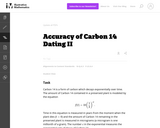Unrestricted Use
CC BY
Rating
0.0 stars

This task is a refinement of ``Carbon 14 dating'' which focuses on accuracy. Because radioactive decay is an atomic process modeled by the laws of quantum mechanics, it is not possible to know with certainty when half of a given quantity of Carbon 14 atoms will decay. This type of question is very important in science and it also provides an opportunity to study the very subtle question of how errors behave when applying a function: in some cases the errors can be magnified while in others they are lessened.

Subject:
Mathematics
Secondary Mathematics
Material Type:
Activity/Lab
Provider:
Illustrative Mathematics
Provider Set:
Illustrative Mathematics
Author:
Illustrative Mathematics
09/13/2012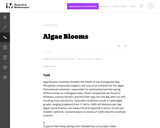Unrestricted Use
CC BY
Rating
0.0 stars

The problem statement describes a changing algae population as reported by the Maryland Department of Natural Resources. In part (a), students are expected to build an exponential function modeling algae concentration from the description given of the relationship between concentrations in cells/ml and days of rapid growth (F-LE.2).

Subject:
Mathematics
Secondary Mathematics
Material Type:
Activity/Lab
Provider:
Illustrative Mathematics
Provider Set:
Illustrative Mathematics
Author:
Illustrative Mathematics
05/01/2012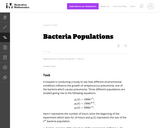Unrestricted Use
CC BY
Rating
0.0 stars

This task provides a real world context for interpreting and solving exponential equations. There are two solutions provided for part (a). The first solution demonstrates how to deduce the conclusion by thinking in terms of the functions and their rates of change. The second approach illustrates a rigorous algebraic demonstration that the two populations can never be equal.

Subject:
Mathematics
Secondary Mathematics
Material Type:
Activity/Lab
Provider:
Illustrative Mathematics
Provider Set:
Illustrative Mathematics
Author:
Illustrative Mathematics
05/01/2012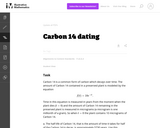Unrestricted Use
CC BY
Rating
0.0 stars

The task requires the student to use logarithms to solve an exponential equation in the realistic context of carbon dating, important in archaeology and geology, among other places. Students should be guided to recognize the use of the natural logarithm when the exponential function has the given base of e, as in this problem. Note that the purpose of this task is algebraic in nature -- closely related tasks exist which approach similar problems from numerical or graphical stances.

Subject:
Mathematics
Secondary Mathematics
Material Type:
Activity/Lab
Provider:
Illustrative Mathematics
Provider Set:
Illustrative Mathematics
Author:
Illustrative Mathematics
05/01/2012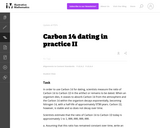Unrestricted Use
CC BY
Rating
0.0 stars

This problem introduces the method used by scientists to date certain organic material. It is based not on the amount of the Carbon 14 isotope remaining in the sample but rather on the ratio of Carbon 14 to Carbon 12. This ratio decreases, hypothetically, at a constant exponential rate as soon as the organic material has ceased to absorb Carbon 14, that is, as soon as it dies. This problem is intended for instructional purposes only. It provides an interesting and important example of mathematical modeling with an exponential function.

Subject:
Mathematics
Secondary Mathematics
Material Type:
Activity/Lab
Provider:
Illustrative Mathematics
Provider Set:
Illustrative Mathematics
Author:
Illustrative Mathematics
08/21/2012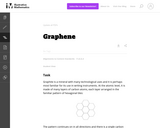Unrestricted Use
CC BY
Rating
0.0 stars

This is a task from the Illustrative Mathematics website that is one part of a complete illustration of the standard to which it is aligned. Each task has at least one solution and some commentary that addresses important asects of the task and its potential use. Here are the first few lines of the commentary for this task: Graphite is a mineral with many technological uses and it is perhaps most familiar for its use in writing instruments. At the atomic level, it is made ...

Subject:
Mathematics
Secondary Mathematics
Material Type:
Activity/Lab
Provider:
Illustrative Mathematics
Provider Set:
Illustrative Mathematics
Author:
Illustrative Mathematics
08/13/2013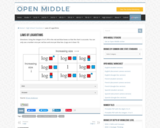Restricted Use
Rating
0.0 stars

Open Middle provides math problems that have a closed beginning, a closed end, and an open middle. This means that there are multiple ways to approach and ultimately solve the problems. Open middle problems generally require a higher Depth of Knowledge than most problems that assess procedural and conceptual understanding.

Subject:
Mathematics
Material Type:
Activity/Lab
Provider:
Open Middle Math
04/26/2022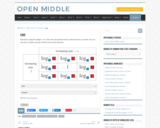Restricted Use
Rating
0.0 stars

Open Middle provides math problems that have a closed beginning, a closed end, and an open middle. This means that there are multiple ways to approach and ultimately solve the problems. Open middle problems generally require a higher Depth of Knowledge than most problems that assess procedural and conceptual understanding.

Subject:
Mathematics
Material Type:
Activity/Lab
Provider:
Open Middle Math
04/26/2022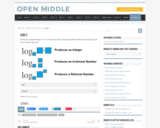Restricted Use
Rating
0.0 stars

Open Middle provides math problems that have a closed beginning, a closed end, and an open middle. This means that there are multiple ways to approach and ultimately solve the problems. Open middle problems generally require a higher Depth of Knowledge than most problems that assess procedural and conceptual understanding.

Subject:
Mathematics
Material Type:
Activity/Lab
Provider:
Open Middle Math
04/26/2022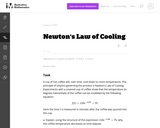Unrestricted Use
CC BY
Rating
0.0 stars

The coffee cooling experiment is a popular example of an exponential model with immediate appeal. The model is realistic and provides a good context for students to practice work with exponential equations.

Subject:
Mathematics
Secondary Mathematics
Material Type:
Activity/Lab
Provider:
Illustrative Mathematics
Provider Set:
Illustrative Mathematics
Author:
Illustrative Mathematics
05/01/2012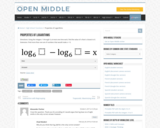Restricted Use
Rating
0.0 stars

Open Middle provides math problems that have a closed beginning, a closed end, and an open middle. This means that there are multiple ways to approach and ultimately solve the problems. Open middle problems generally require a higher Depth of Knowledge than most problems that assess procedural and conceptual understanding.

Subject:
Mathematics
Material Type:
Activity/Lab
Provider:
Open Middle Math
04/26/2022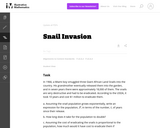Unrestricted Use
CC BY
Rating
0.0 stars

The purpose of this task is to give students experience modeling a real-world example of exponential growth, in a context that provides a vivid illustration of the power of exponential growth, for example the cost of inaction for a year.

Subject:
Mathematics
Secondary Mathematics
Material Type:
Activity/Lab
Provider:
Illustrative Mathematics
Provider Set:
Illustrative Mathematics
Author:
Illustrative Mathematics
05/01/2012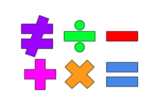Unrestricted Use
Public Domain
Rating
0.0 stars

This lesson is for a math classroom, but can be adapted to fit any grade, subject, or content.&nbsp;&nbsp;In this lesson, students will use an iPad and its features: Keynote, Pages, Garageband, Numbers, Presentation, and iMovie.&nbsp; Students will use 3 of those features/programs to create a video lesson consisting of several examples from a topic of their choice.&nbsp; This project is in place of a term final, so their chosen topic should be from their current term.Image citation: The image is one I created.&nbsp;&nbsp;

Subject:
Secondary Mathematics
Material Type:
Assessment
Author:
Tyrani Bevell